# SSAT Upper Level Math : Equilateral Triangles

## Example Questions

← Previous 1 3 4

### Example Question #58 : Properties Of Triangles

An equilateral triangle has a perimeter of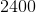units. What is the length of each side?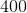units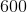units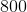unitsunitsunits

Explanation:

Because an equilateral triangle has three sides that are the same length, divide the given perimeter by 3 to find the length of each side.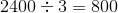### Example Question #1 : Equilateral Triangles

The perimeter of a equilateral triangle is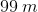. In meters, what is the length of a side of this triangle?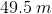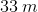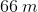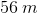Explanation:

Since all three sides are equal in an equilateral triangle, we can just divide the perimeter by 3 to find a side length.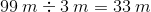### Example Question #1 : How To Find The Length Of The Side Of An Equilateral Triangle

The perimeter of an equilateral triangle is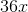. What is the length of a side of this triangle?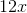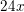Explanation:

Because all the sides in an equilateral triangle are equal, we can just divide the perimeter by 3 to find the length of a side.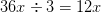### Example Question #1 : How To Find The Length Of The Side Of An Equilateral Triangle

The perimeter of an equilateral triangle is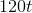. What is the length of one side of the triangle?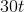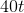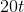Explanation:

Since an equilateral triangle has sides that are all the same, divide the perimeter byto get the length of each side.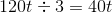### Example Question #64 : Properties Of Triangles

The perimeter of an equilateral triangle is 39 meters. In meters, how long is one side of the triangle?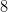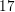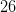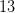Explanation:

An equilateral triangle has sides that are the same length. Then, to find the length of one side, you only need to divide the perimeter by 3.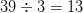### Example Question #65 : Properties Of Triangles

The perimeter of an equilateral triangle is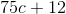. What is the length of one side of this triangle?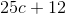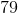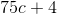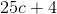Explanation:

Since an equilateral triangle has three equal sides, divide the perimeter byto find the length of each side.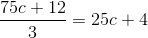### Example Question #66 : Properties Of Triangles

The perimeter of an equilateral triangle is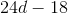. What is the length of one side of this triangle?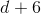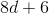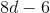Explanation:

Since an equilateral triangle has three equal sides, divide the perimeter byto find the length of one side.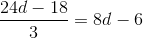### Example Question #1 : Equilateral Triangles

What is the difference between an equilateral triangle and a scalene triangle?

Their color

The number of side lengths

The length of their sides

The sum of their angle measurements

The sum of their side lengths

The length of their sides

Explanation:

Of the choices listed, the main difference between an equilateral triangle and a scalene triangle is their side lengths. An equilateral triangle has to haveequal sides, but a scalene triangle can have all different side lengths.

### Example Question #61 : Properties Of Triangles

An equilateral triangle is circumscribed about a circle of radius 16. Give the area of the triangle.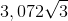The correct answer is not among the other choices.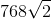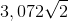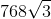Explanation:

The circle and triangle referenced are below, along with a radius to one side and a segment to one vertex: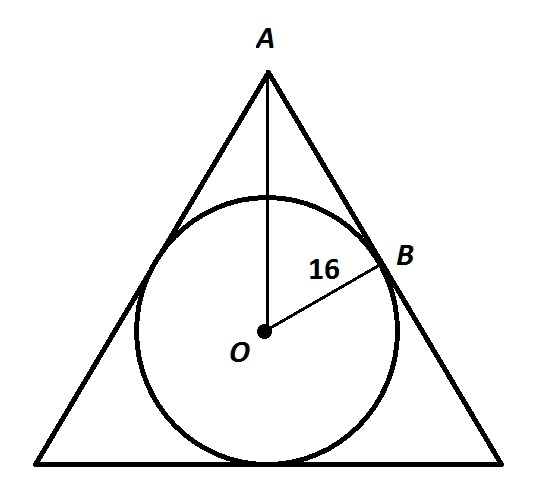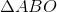is a 30-60-90 triangle, so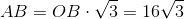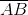is one-half of a side of the triangle, so the sidelength is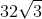. The area of the triangle is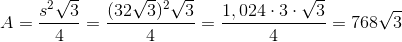### Example Question #1 : How To Find The Area Of An Equilateral Triangle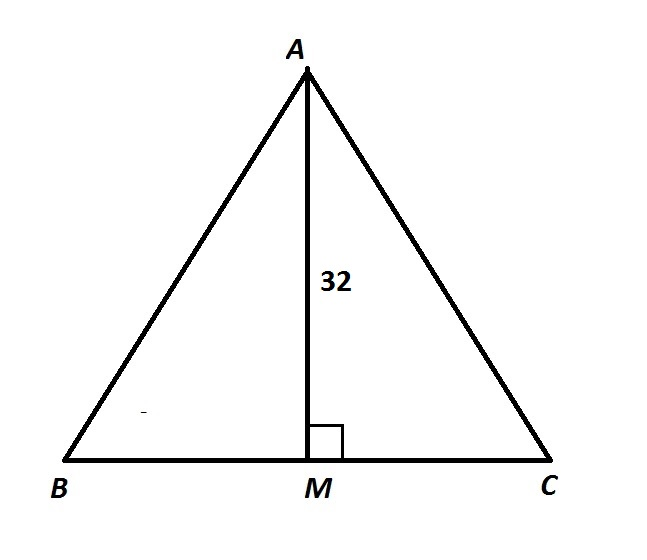In the above diagram,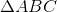is equilateral. Give its area.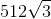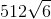The correct answer is not among the other responses.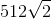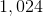The correct answer is not among the other responses.

Explanation:

The interior angles of an equilateral triangle all measure 60 degrees, so, by the 30-60-90 Theorem,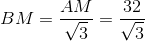Also,is the midpoint of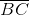, so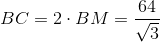; this is the base.

The area of this triangle is half the product of the base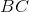and the height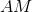: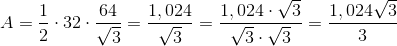This answer is not among the given choices.

← Previous 1 3 4

### All SSAT Upper Level Math Resources